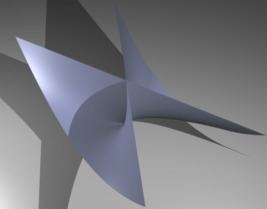# Pinch point (mathematics)

Updated on
Covid-19In geometry, a pinch point or cuspidal point is a type of singular point on an algebraic surface.

The equation for the surface near a pinch point may be put in the form

f ( u , v , w ) = u 2 v w 2 + [ 4 ]

where  denotes terms of degree 4 or more and v is not a square in the ring of functions.

For example the surface 1 2 x + x 2 y z 2 = 0 near the point ( 1 , 0 , 0 ) , meaning in coordinates vanishing at that point, has the form above. In fact, if u = 1 x , v = y and w = z then { u , v , w } is a system of coordinates vanishing at ( 1 , 0 , 0 ) then 1 2 x + x 2 y z 2 = ( 1 x ) 2 y z 2 = u 2 v w 2 is written in the canonical form.

The simplest example of a pinch point is the hypersurface defined by the equation u 2 v w 2 = 0 called Whitney umbrella.

The pinch point (in this case the origin) is a limit of normal crossings singular points (the v -axis in this case). These singular points are intimately related in the sense that in order to resolve the pinch point singularity one must blow-up the whole v -axis and not only the pinch point.

Topics